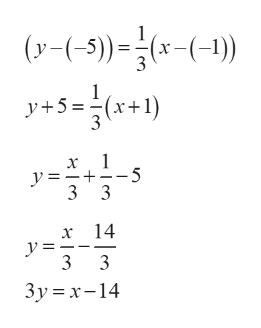# Graph the line with the slope 1/3 passing through the point (-1,-5)

Question
6 views

Graph the line with the slope 1/3 passing through the point (-1,-5)

check_circle

star
star
star
star
star
1 Rating
Step 1

Given, the slope 1/3 and a point (-1,-5)

The equation of line with slope m passing through the point (x1, y1) is given by,

(y - y1) = m(x - x1)

Here, x1 = -1,  y1 = -5 and m = 1/3

Then the equation of the given line is,

...help_outlineImage Transcriptioncloser(-5)-(-) y+5=(x+1) -(-1) 5 y=_+ 3 3 14 x y= 3 3 3y x-14 fullscreen

### Want to see the full answer?

See Solution

#### Want to see this answer and more?

Solutions are written by subject experts who are available 24/7. Questions are typically answered within 1 hour.*

See Solution
*Response times may vary by subject and question.
Tagged in

### Other